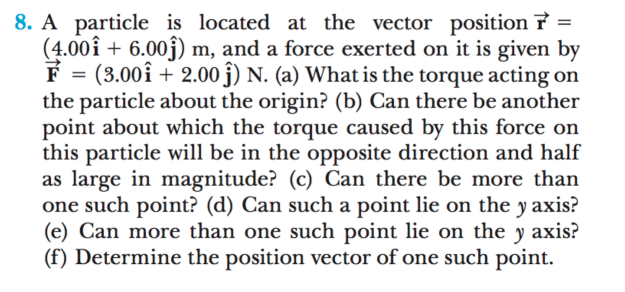# 8. A particle is located at the vector position 7 =(4.00î + 6.00j) m, and a force exerted on it is given byF = (3.00î + 2.00 j) N. (a) What is the torque acting onthe particle about the origin? (b) Can there be anotherpoint about which the torque caused by this force onthis particle will be in the opposite direction and halfas large in magnitude? (c) Can there be more thanone such point? (d) Can such a point lie on the y axis?(e) Can more than one such point lie on the y axis?(f) Determine the position vector of one such point.%3D

Question
1 viewshelp_outlineImage Transcriptionclose8. A particle is located at the vector position 7 = (4.00î + 6.00j) m, and a force exerted on it is given by F = (3.00î + 2.00 j) N. (a) What is the torque acting on the particle about the origin? (b) Can there be another point about which the torque caused by this force on this particle will be in the opposite direction and half as large in magnitude? (c) Can there be more than one such point? (d) Can such a point lie on the y axis? (e) Can more than one such point lie on the y axis? (f) Determine the position vector of one such point. %3D fullscreen
check_circle

Step 1

Given that

Step 2

a)

The torque on the particle is,

Step 3

b)

Here the force remains the same, but the magnitude of the to...

### Want to see the full answer?

See Solution

#### Want to see this answer and more?

Solutions are written by subject experts who are available 24/7. Questions are typically answered within 1 hour.*

See Solution
*Response times may vary by subject and question.
Tagged in

### Physics